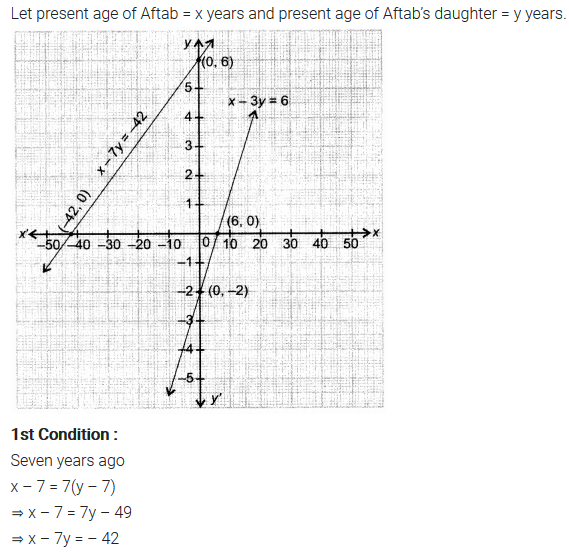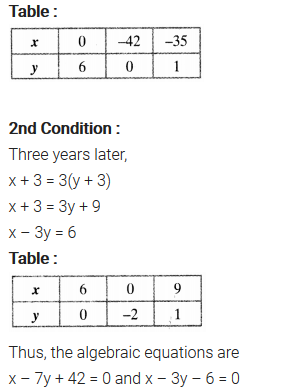NCERT Solutions for Class 10 Maths Chapter 3 Ex 3.1 | Monster Thinks - Monster Thinks

# NCERT Solutions for Class 10 Maths Chapter 3 Pair of Linear Equations in Two Variables Ex 3.1

Get Free NCERT Solutions for Class 10 Maths Chapter 3 Ex 3.1. Pair of Linear Equations in Two Variables Class 10 Maths NCERT Solutions are extremely helpful while doing your homework. Exercise 3.1 Class 10 Maths NCERT Solutions were prepared by Experienced MonsterThinks.in Teachers. Detailed answers of  Class 10 Pair of Linear Equations in Two Variables Exercise 3.1 provided in NCERT TextBook.

Topics and Sub Topics in Class 10 Maths Chapter 3 Pair of Linear Equations in Two Variables:

 Section Name Topic Name 3 Pair of Linear Equations in Two Variables 3.1 Introduction 3.2 Pair Of Linear Equations In Two Variables 3.3 Graphical Method Of Solution Of A Pair Of Linear Equations 3.4 Algebraic Methods Of Solving A Pair Of Linear Equations 3.4.1 Substitution Method 3.4.2 Elimination Method 3.4.3 Cross-Multiplication Method 3.5 Equations Reducible To A Pair Of Linear Equations In Two Variables 3.6 Summary

NCERT Solutions for Class 10 Maths Chapter 3 Pair of Linear Equations in Two Variables Ex 3.1

NCERT Solutions for Class 10 Maths Chapter 3 Pair of Linear Equations in Two Variables Ex 3.1 are part of NCERT Solutions for Class 10 Maths. Here we have given NCERT Solutions for Class 10 Maths Chapter 3 Pair of Linear Equations in Two Variables Exercise 3.1

 Board CBSE Textbook NCERT Class Class 10 Subject Maths Chapter Chapter 3 Chapter Name Pair of Linear Equations in Two Variables Exercise Ex 3.1 Number of Questions Solved 3 Category NCERT Solutions

You can also download the free PDF of  Ex 3.1 Class 10 Pair of Linear Equations in Two Variables NCERT Solutions or save the solution images and take the printout to keep it handy for your exam preparation.

Ex 3.1 Class 10 Maths Question 1.
Aftab tells his daughter, “Seven years ago, I was seven times as old as you were then. Also, three years from now, I shall be three times as old as you will be”. Isn’t this interesting? Represent this situation algebraically and graphically.
Solution:Ex 3.1 Class 10 Maths Question 2.
The coach of a cricket team buys 3 bats and 6 balls for ₹ 3900. Later, she buys another bat and 3 more balls of the same kind for ₹1300. Represent this situation algebraically and geometrically.
Solution:Ex 3.1 Class 10 Maths Question 3.
The cost of 2 kg of apples and 1 kg of grapes on a day was found to be ₹160.  After a month, the cost of 4 kg of apples and 2 kg of grapes is ₹300. Represent the situation algebraically and geometrically.
Solution:CBSE NCERT Solutions of Chapter 1